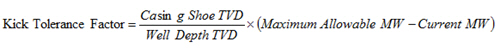## Kick Tolerance Factor Calculation

The following formula is the kick tolerance factor.Where;

Casing shoe TVD in ft

Well depth TVD in ft

Maximum Allowable MW in ppg

Current MW in ppg

For more understanding, let’s try to calculate the kick tolerance factor (KTF) using the following information: